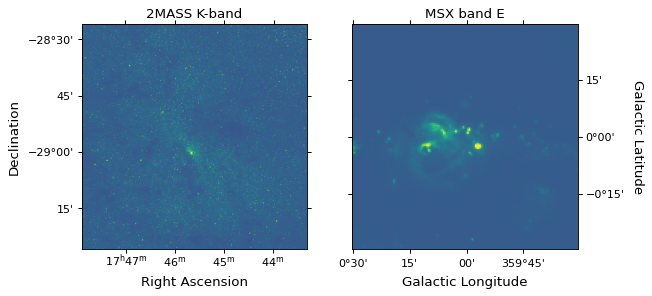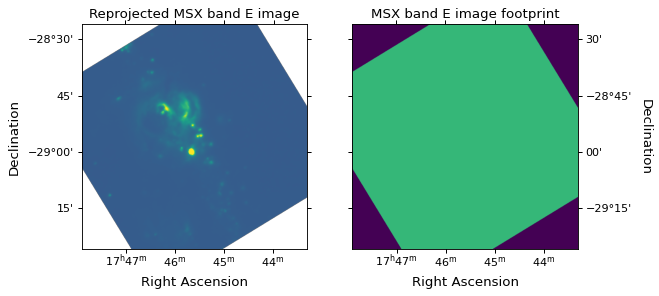# Image reprojection (resampling)¶

## Introduction¶

The reproject package implements image reprojection (resampling) methods for astronomical images and more generally n-dimensional data. These assume that the WCS information contained in the data are correct. This package does not do image registration, which is the process of aligning images where one or more images may have incorrect or missing WCS.

You can install reproject with pip:

```pip install reproject
```

or with conda:

```conda install -c astropy reproject
```

More detailed installation instructions can be found in Installing reproject.

## Quick start¶

A common use case is that you have two FITS images, and want to reproject one to the same header as the other. This can easily be done with the reproject package, and we demonstrate this in the following example. We start off by downloading two example images from http://data.astropy.org, namely a 2MASS K-band image and an MSX band E image of the Galactic center:

```from astropy.io import fits
from astropy.utils.data import get_pkg_data_filename
hdu1 = fits.open(get_pkg_data_filename('galactic_center/gc_2mass_k.fits'))
hdu2 = fits.open(get_pkg_data_filename('galactic_center/gc_msx_e.fits'))
```

We can examine the two images (this makes use of the wcsaxes package behind the scenes):

```from astropy.wcs import WCS
import matplotlib.pyplot as plt

ax1.imshow(hdu1.data, origin='lower', vmin=-100., vmax=2000.)
ax1.coords['ra'].set_axislabel('Right Ascension')
ax1.coords['dec'].set_axislabel('Declination')
ax1.set_title('2MASS K-band')

ax2.imshow(hdu2.data, origin='lower', vmin=-2.e-4, vmax=5.e-4)
ax2.coords['glon'].set_axislabel('Galactic Longitude')
ax2.coords['glat'].set_axislabel('Galactic Latitude')
ax2.coords['glat'].set_axislabel_position('r')
ax2.coords['glat'].set_ticklabel_position('r')
ax2.set_title('MSX band E')
```

(png, svg, pdf)We now reproject the MSX image to be in the same projection as the 2MASS image:

```from reproject import reproject_interp
```

The `reproject_interp()` function above returns the reprojected array as well as an array that provides information on the footprint of the first image in the new reprojected image plane (essentially which pixels in the new image had a corresponding pixel in the old image). We can now visualize the reprojected data and footprint:

```from astropy.wcs import WCS
import matplotlib.pyplot as plt

ax1.imshow(array, origin='lower', vmin=-2.e-4, vmax=5.e-4)
ax1.coords['ra'].set_axislabel('Right Ascension')
ax1.coords['dec'].set_axislabel('Declination')
ax1.set_title('Reprojected MSX band E image')

ax2.imshow(footprint, origin='lower', vmin=0, vmax=1.5)
ax2.coords['ra'].set_axislabel('Right Ascension')
ax2.coords['dec'].set_axislabel('Declination')
ax2.coords['dec'].set_axislabel_position('r')
ax2.coords['dec'].set_ticklabel_position('r')
ax2.set_title('MSX band E image footprint')
```

(png, svg, pdf)We can then write out the image to a new FITS file. Note that, as for plotting, we can use the header from the 2MASS image since both images are now in the same projection:

```fits.writeto('msx_on_2mass_header.fits', array, hdu1.header, overwrite=True)
```

The reproject package supports a number of different algorithms for reprojection (interpolation, flux-conserving reprojection, etc.) and different types of data (images, spectral cubes, HEALPIX images, etc.). For more information, we encourage you to read the full documentation below!

## Documentation¶

The reproject package consists of a few high-level functions to do reprojection using different algorithms, which depend on the type of data that you want to reproject.

## Reference/API¶

### reproject Package¶

Astropy affiliated package for image reprojection (resampling).

#### Functions¶

 `reproject_adaptive`(input_data, output_projection) Reproject celestial slices from an 2d array from one WCS to another using the DeForest (2004) adaptive resampling algorithm. `reproject_exact`(input_data, output_projection) Reproject data to a new projection using flux-conserving spherical polygon intersection (this is the slowest algorithm). `reproject_from_healpix`(input_data, …[, …]) Reproject data from a HEALPIX projection to a standard projection. `reproject_interp`(input_data, output_projection) Reproject data to a new projection using interpolation (this is typically the fastest way to reproject an image). `reproject_to_healpix`(input_data, …[, …]) Reproject data from a standard projection to a HEALPIX projection. `test`(**kwargs) Run the tests for the package.

### reproject.mosaicking Package¶

#### Functions¶

 `find_optimal_celestial_wcs`(input_data[, …]) Given one or more images, return an optimal WCS projection object and shape. `reproject_and_coadd`(input_data, …[, …]) Given a set of input images, reproject and co-add these to a single final image.